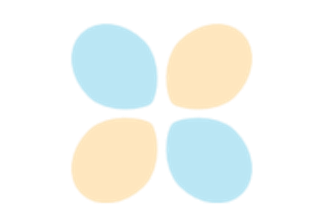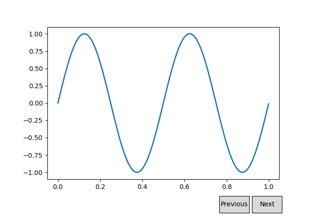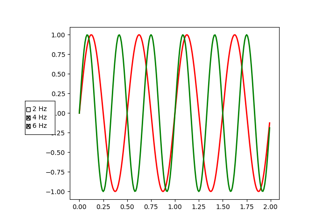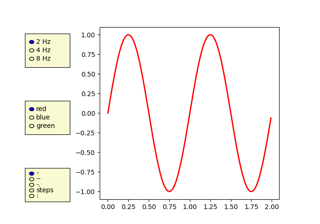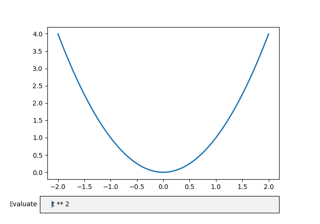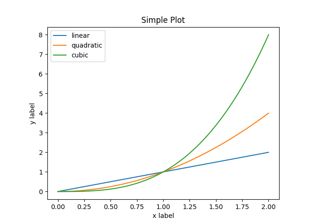# matplotlib.pyplot.draw¶

matplotlib.pyplot.draw()[source]

Redraw the current figure.

This is used to update a figure that has been altered, but not automatically re-drawn. If interactive mode is on (via ion()), this should be only rarely needed, but there may be ways to modify the state of a figure without marking it as "stale". Please report these cases as bugs.

This is equivalent to calling fig.canvas.draw_idle(), where fig is the current figure.

## Examples using matplotlib.pyplot.draw¶